Skip to content →

# Tag: Quillen

Last time we discovered that the mental picture to view prime numbers as knots in $S^3$ was first dreamed up by David Mumford. Today, we’ll focus on where and when this happened.

3. When did Mazur write his unpublished preprint?

According to his own website, Barry Mazur did write the paper Remarks on the Alexander polynomial in 1963 or 1964. A quick look at the references gives us a coarse lower- and upper-estimate.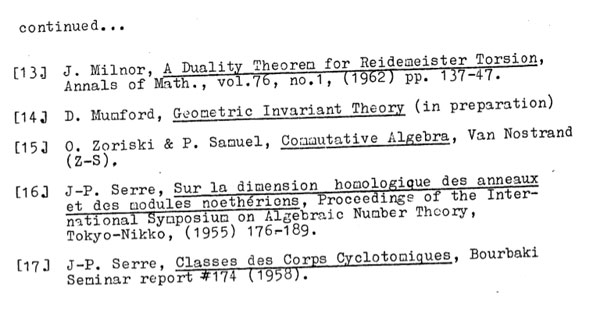Apart from a paper by Iwasawa and one by Milnor, all references predate 1962 giving us a lower-bound. More interesting is reference (14) to David Mumford’s Geometric Invariant Theory (GIT) which was first published in 1965 and is referred to as ‘in preparation’, so the paper was written no later than 1965. If we look a bit closer we see than some GIT-references are very preciseindicating that Mazur must have had the final version of GIT to consult, making it rather difficult to believe that the preprint was written late 1963 or early 1964.

Mazur’s dating of the preprint is probably based on this penciled note on the frontpage of the only surviving copy of the preprintIt reads : “Date from about 63/64, H.R. Morton”. Hugh Morton of Liverpool University confirms that it is indeed his writing on the preprint.

Further, he told me that early 64 Christopher Zeeman held a Topology Symposium in Cambridge UK, where Hugh was a graduate student at the time and, as far as he could recall, Mazur attended that conference and gave him the preprint on that occasion, whence the 63/64 dating. Hugh kindly offered to double-check this with Terry Wall who cannot remember Mazur attending that particular conference.

In fact, we will see that a more correct dating of the Mazur-preprint will be : late 1964 or early 1965.

4. The birthday : July 10th 1964

Clearly, Mumford’s insight predates the Mazur-preprint. In the first section, Mazur mentions ‘Grothendieck cohomology groups’ rather than ‘Etale cohomology groups’.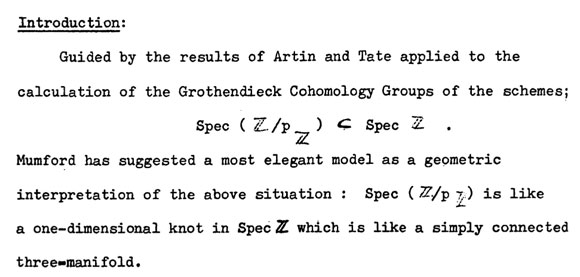At the time, Artin’s seminar notes on Grothendieck topologies (spring 1962) were widely distributed, and Artin and Grothendieck were in the process of developing etale cohomology in their Paris 1963/64 seminar SGA 4, while Mumford was working on GIT in Harvard.Mike Artin, David Mumford and Jean-Louis Verdier all attended the Woods Hole conference from july 6 till july 31 1964, famous for producing the Atiyah-Bott fixed point theorem (according to Fulton first proved by Verdier at the conference).

Etale cohomology was a hot topic at that conference. On july 10th there were three talks, Artin spoke on ‘Etale cohomology of schemes’, Verdier on ‘A duality theorem in the etale cohomology of schemes’ and John Tate on ‘Etale cohomology over number fields’.

After a first week of talks, more informal seminars were organized, including the Atiyah-Bott seminar leading to the ‘Woods hole duality theorem’ and one by Lubin-Tate and Serre on elliptic curves and formal groups. Two seminars adressed Etale Cohomology.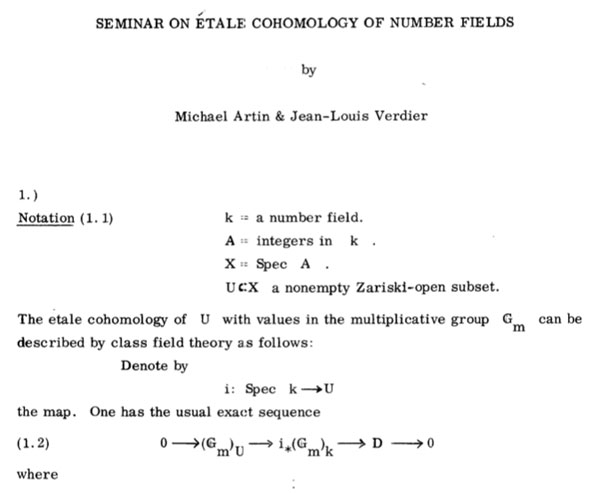Artin and Verdier ran a seminar on the etale cohomology of number fields leading to their duality result, and, three young turks : Daniel Quillen, Steve Kleiman and Robin Hartshorne ran a Baby Seminar on Etale cohomology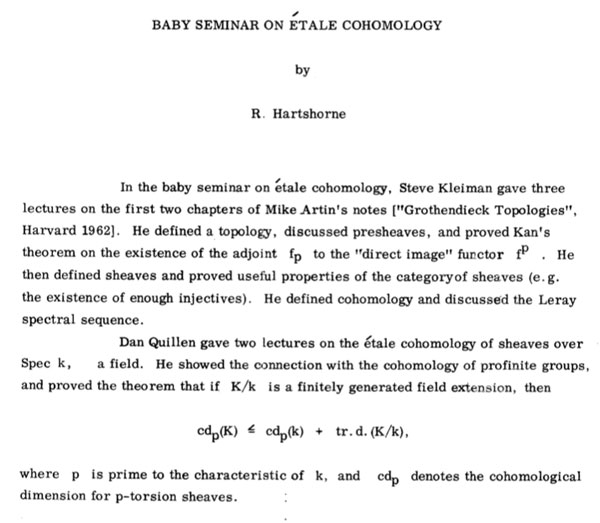Probably it is safe to say that the talks by Artin, Verdier and Tate on July 10th sparked the primes=knots idea, and if not then, a couple of days later.

5. The birthplace : the Whitney Estate

The ‘Woods Hole’ conference took place at the Whitney Estate and all the lectures took place in the rustic rooms of the main building and the participants (and their families) were housed in rented cottages in the neighborhood, for the duration of the summer.

The only picture i managed to find from the Whitney house comes from a rather surprising source : Gardeners and Caretakers ofWoods Hole. Anyway, here it is :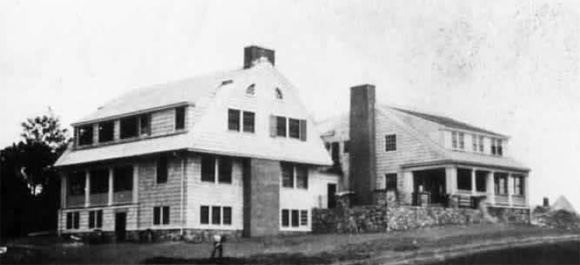Probably, the knots=primes analogy was first dreamed up inside, or in the immediate neighborhood, on a walk to or from the cottages, overlooking the harbor.

The Monster is the largest of the 26 sporadic simple groups and has order

808 017 424 794 512 875 886 459 904 961 710 757 005 754 368 000 000 000

= 2^46 3^20 5^9 7^6 11^2 13^3 17 19 23 29 31 41 47 59 71.

It is not so much the size of its order that makes it hard to do actual calculations in the monster, but rather the dimensions of its smallest non-trivial irreducible representations (196 883 for the smallest, 21 296 876 for the next one, and so on).

In characteristic two there is an irreducible representation of one dimension less (196 882) which appears to be of great use to obtain information. For example, Robert Wilson used it to prove that The Monster is a Hurwitz group. This means that the Monster is generated by two elements g and h satisfying the relations

$g^2 = h^3 = (gh)^7 = 1$

Geometrically, this implies that the Monster is the automorphism group of a Riemann surface of genus g satisfying the Hurwitz bound 84(g-1)=#Monster. That is,

g=9619255057077534236743570297163223297687552000000001=42151199 * 293998543 * 776222682603828537142813968452830193

Or, in analogy with the Klein quartic which can be constructed from 24 heptagons in the tiling of the hyperbolic plane, there is a finite region of the hyperbolic plane, tiled with heptagons, from which we can construct this monster curve by gluing the boundary is a specific way so that we get a Riemann surface with exactly 9619255057077534236743570297163223297687552000000001 holes. This finite part of the hyperbolic tiling (consisting of #Monster/7 heptagons) we’ll call the empire of the monster and we’d love to describe it in more detail.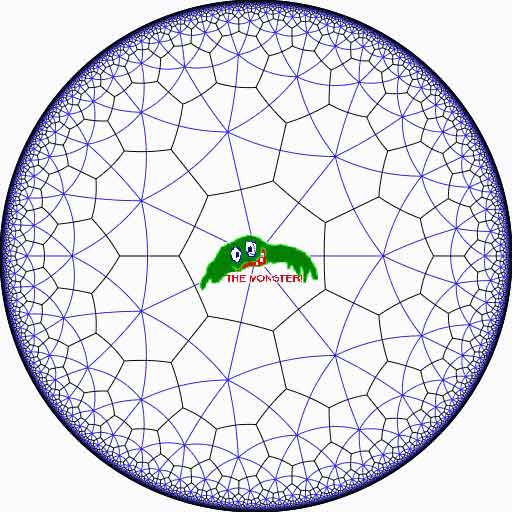Look at the half-edges of all the heptagons in the empire (the picture above learns that every edge in cut in two by a blue geodesic). They are exactly #Monster such half-edges and they form a dessin d’enfant for the monster-curve.

If we label these half-edges by the elements of the Monster, then multiplication by g in the monster interchanges the two half-edges making up a heptagonal edge in the empire and multiplication by h in the monster takes a half-edge to the one encountered first by going counter-clockwise in the vertex of the heptagonal tiling. Because g and h generated the Monster, the dessin of the empire is just a concrete realization of the monster.

Because g is of order two and h is of order three, the two permutations they determine on the dessin, gives a group epimorphism $C_2 \ast C_3 = PSL_2(\mathbb{Z}) \rightarrow \mathbb{M}$ from the modular group $PSL_2(\mathbb{Z})$ onto the Monster-group.

In noncommutative geometry, the group-algebra of the modular group $\mathbb{C} PSL_2$ can be interpreted as the coordinate ring of a noncommutative manifold (because it is formally smooth in the sense of Kontsevich-Rosenberg or Cuntz-Quillen) and the group-algebra of the Monster $\mathbb{C} \mathbb{M}$ itself corresponds in this picture to a finite collection of ‘points’ on the manifold. Using this geometric viewpoint we can now ask the question What does the Monster see of the modular group?

To make sense of this question, let us first consider the commutative equivalent : what does a point P see of a commutative variety X?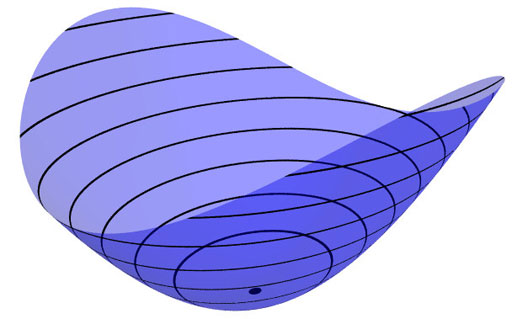Evaluation of polynomial functions in P gives us an algebra epimorphism $\mathbb{C}[X] \rightarrow \mathbb{C}$ from the coordinate ring of the variety $\mathbb{C}[X]$ onto $\mathbb{C}$ and the kernel of this map is the maximal ideal $\mathfrak{m}_P$ of
$\mathbb{C}[X]$ consisting of all functions vanishing in P.

Equivalently, we can view the point $P= \mathbf{spec}~\mathbb{C}[X]/\mathfrak{m}_P$ as the scheme corresponding to the quotient $\mathbb{C}[X]/\mathfrak{m}_P$. Call this the 0-th formal neighborhood of the point P.

This sounds pretty useless, but let us now consider higher-order formal neighborhoods. Call the affine scheme $\mathbf{spec}~\mathbb{C}[X]/\mathfrak{m}_P^{n+1}$ the n-th forml neighborhood of P, then the first neighborhood, that is with coordinate ring $\mathbb{C}[X]/\mathfrak{m}_P^2$ gives us tangent-information. Alternatively, it gives the best linear approximation of functions near P.
The second neighborhood $\mathbb{C}[X]/\mathfrak{m}_P^3$ gives us the best quadratic approximation of function near P, etc. etc.

These successive quotients by powers of the maximal ideal $\mathfrak{m}_P$ form a system of algebra epimorphisms

$\ldots \frac{\mathbb{C}[X]}{\mathfrak{m}_P^{n+1}} \rightarrow \frac{\mathbb{C}[X]}{\mathfrak{m}_P^{n}} \rightarrow \ldots \ldots \rightarrow \frac{\mathbb{C}[X]}{\mathfrak{m}_P^{2}} \rightarrow \frac{\mathbb{C}[X]}{\mathfrak{m}_P} = \mathbb{C}$

and its inverse limit $\underset{\leftarrow}{lim}~\frac{\mathbb{C}[X]}{\mathfrak{m}_P^{n}} = \hat{\mathcal{O}}_{X,P}$ is the completion of the local ring in P and contains all the infinitesimal information (to any order) of the variety X in a neighborhood of P. That is, this completion $\hat{\mathcal{O}}_{X,P}$ contains all information that P can see of the variety X.

In case P is a smooth point of X, then X is a manifold in a neighborhood of P and then this completion
$\hat{\mathcal{O}}_{X,P}$ is isomorphic to the algebra of formal power series $\mathbb{C}[[ x_1,x_2,\ldots,x_d ]]$ where the $x_i$ form a local system of coordinates for the manifold X near P.

Right, after this lengthy recollection, back to our question what does the monster see of the modular group? Well, we have an algebra epimorphism

$\pi~:~\mathbb{C} PSL_2(\mathbb{Z}) \rightarrow \mathbb{C} \mathbb{M}$

and in analogy with the commutative case, all information the Monster can gain from the modular group is contained in the $\mathfrak{m}$-adic completion

$\widehat{\mathbb{C} PSL_2(\mathbb{Z})}_{\mathfrak{m}} = \underset{\leftarrow}{lim}~\frac{\mathbb{C} PSL_2(\mathbb{Z})}{\mathfrak{m}^n}$

where $\mathfrak{m}$ is the kernel of the epimorphism $\pi$ sending the two free generators of the modular group $PSL_2(\mathbb{Z}) = C_2 \ast C_3$ to the permutations g and h determined by the dessin of the pentagonal tiling of the Monster’s empire.

As it is a hopeless task to determine the Monster-empire explicitly, it seems even more hopeless to determine the kernel $\mathfrak{m}$ let alone the completed algebra… But, (surprise) we can compute $\widehat{\mathbb{C} PSL_2(\mathbb{Z})}_{\mathfrak{m}}$ as explicitly as in the commutative case we have $\hat{\mathcal{O}}_{X,P} \simeq \mathbb{C}[[ x_1,x_2,\ldots,x_d ]]$ for a point P on a manifold X.

Here the details : the quotient $\mathfrak{m}/\mathfrak{m}^2$ has a natural structure of $\mathbb{C} \mathbb{M}$-bimodule. The group-algebra of the monster is a semi-simple algebra, that is, a direct sum of full matrix-algebras of sizes corresponding to the dimensions of the irreducible monster-representations. That is,

$\mathbb{C} \mathbb{M} \simeq \mathbb{C} \oplus M_{196883}(\mathbb{C}) \oplus M_{21296876}(\mathbb{C}) \oplus \ldots \ldots \oplus M_{258823477531055064045234375}(\mathbb{C})$

with exactly 194 components (the number of irreducible Monster-representations). For any $\mathbb{C} \mathbb{M}$-bimodule $M$ one can form the tensor-algebra

$T_{\mathbb{C} \mathbb{M}}(M) = \mathbb{C} \mathbb{M} \oplus M \oplus (M \otimes_{\mathbb{C} \mathbb{M}} M) \oplus (M \otimes_{\mathbb{C} \mathbb{M}} M \otimes_{\mathbb{C} \mathbb{M}} M) \oplus \ldots \ldots$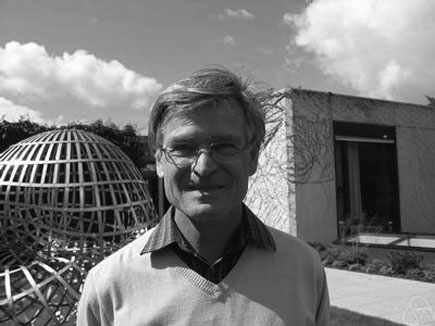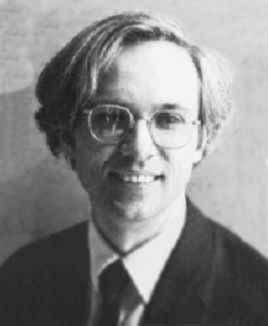and applying the formal neighborhood theorem for formally smooth algebras (such as $\mathbb{C} PSL_2(\mathbb{Z})$) due to Joachim Cuntz (left) and Daniel Quillen (right) we have an isomorphism of algebras

$\widehat{\mathbb{C} PSL_2(\mathbb{Z})}_{\mathfrak{m}} \simeq \widehat{T_{\mathbb{C} \mathbb{M}}(\mathfrak{m}/\mathfrak{m}^2)}$

where the right-hand side is the completion of the tensor-algebra (at the unique graded maximal ideal) of the $\mathbb{C} \mathbb{M}$-bimodule $\mathfrak{m}/\mathfrak{m}^2$, so we’d better describe this bimodule explicitly.

Okay, so what’s a bimodule over a semisimple algebra of the form $S=M_{n_1}(\mathbb{C}) \oplus \ldots \oplus M_{n_k}(\mathbb{C})$? Well, a simple S-bimodule must be either (1) a factor $M_{n_i}(\mathbb{C})$ with all other factors acting trivially or (2) the full space of rectangular matrices $M_{n_i \times n_j}(\mathbb{C})$ with the factor $M_{n_i}(\mathbb{C})$ acting on the left, $M_{n_j}(\mathbb{C})$ acting on the right and all other factors acting trivially.

That is, any S-bimodule can be represented by a quiver (that is a directed graph) on k vertices (the number of matrix components) with a loop in vertex i corresponding to each simple factor of type (1) and a directed arrow from i to j corresponding to every simple factor of type (2).

That is, for the Monster, the bimodule $\mathfrak{m}/\mathfrak{m}^2$ is represented by a quiver on 194 vertices and now we only have to determine how many loops and arrows there are at or between vertices.

Using Morita equivalences and standard representation theory of quivers it isn’t exactly rocket science to determine that the number of arrows between the vertices corresponding to the irreducible Monster-representations $S_i$ and $S_j$ is equal to

$dim_{\mathbb{C}}~Ext^1_{\mathbb{C} PSL_2(\mathbb{Z})}(S_i,S_j)-\delta_{ij}$

Now, I’ve been wasting a lot of time already here explaining what representations of the modular group have to do with quivers (see for example here or some other posts in the same series) and for quiver-representations we all know how to compute Ext-dimensions in terms of the Euler-form applied to the dimension vectors.

Right, so for every Monster-irreducible $S_i$ we have to determine the corresponding dimension-vector $~(a_1,a_2;b_1,b_2,b_3)$ for the quiver

$\xymatrix{ & & & & \vtx{b_1} \\ \vtx{a_1} \ar[rrrru]^(.3){B_{11}} \ar[rrrrd]^(.3){B_{21}} \ar[rrrrddd]_(.2){B_{31}} & & & & \\ & & & & \vtx{b_2} \\ \vtx{a_2} \ar[rrrruuu]_(.7){B_{12}} \ar[rrrru]_(.7){B_{22}} \ar[rrrrd]_(.7){B_{23}} & & & & \\ & & & & \vtx{b_3}}$

Now the dimensions $a_i$ are the dimensions of the +/-1 eigenspaces for the order 2 element g in the representation and the $b_i$ are the dimensions of the eigenspaces for the order 3 element h. So, we have to determine to which conjugacy classes g and h belong, and from Wilson’s paper mentioned above these are classes 2B and 3B in standard Atlas notation.

So, for each of the 194 irreducible Monster-representations we look up the character values at 2B and 3B (see below for the first batch of those) and these together with the dimensions determine the dimension vector $~(a_1,a_2;b_1,b_2,b_3)$.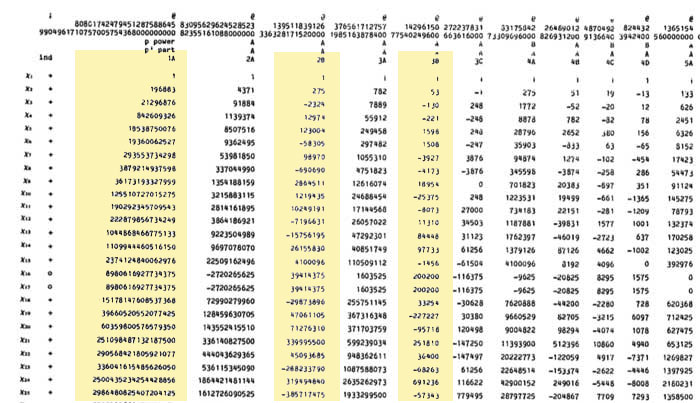For example take the 196883-dimensional irreducible. Its 2B-character is 275 and the 3B-character is 53. So we are looking for a dimension vector such that $a_1+a_2=196883, a_1-275=a_2$ and $b_1+b_2+b_3=196883, b_1-53=b_2=b_3$ giving us for that representation the dimension vector of the quiver above $~(98579,98304,65663,65610,65610)$.

Okay, so for each of the 194 irreducibles $S_i$ we have determined a dimension vector $~(a_1(i),a_2(i);b_1(i),b_2(i),b_3(i))$, then standard quiver-representation theory asserts that the number of loops in the vertex corresponding to $S_i$ is equal to

$dim(S_i)^2 + 1 – a_1(i)^2-a_2(i)^2-b_1(i)^2-b_2(i)^2-b_3(i)^2$

and that the number of arrows from vertex $S_i$ to vertex $S_j$ is equal to

$dim(S_i)dim(S_j) – a_1(i)a_1(j)-a_2(i)a_2(j)-b_1(i)b_1(j)-b_2(i)b_2(j)-b_3(i)b_3(j)$

This data then determines completely the $\mathbb{C} \mathbb{M}$-bimodule $\mathfrak{m}/\mathfrak{m}^2$ and hence the structure of the completion $\widehat{\mathbb{C} PSL_2}_{\mathfrak{m}}$ containing all information the Monster can gain from the modular group.

But then, one doesn’t have to go for the full regular representation of the Monster. Any faithful permutation representation will do, so we might as well go for the one of minimal dimension.

That one is known to correspond to the largest maximal subgroup of the Monster which is known to be a two-fold extension $2.\mathbb{B}$ of the Baby-Monster. The corresponding permutation representation is of dimension 97239461142009186000 and decomposes into Monster-irreducibles

$S_1 \oplus S_2 \oplus S_4 \oplus S_5 \oplus S_9 \oplus S_{14} \oplus S_{21} \oplus S_{34} \oplus S_{35}$

(in standard Atlas-ordering) and hence repeating the arguments above we get a quiver on just 9 vertices! The actual numbers of loops and arrows (I forgot to mention this, but the quivers obtained are actually symmetric) obtained were found after laborious computations mentioned in this post and the details I’ll make avalable here.

Anyone who can spot a relation between the numbers obtained and any other part of mathematics will obtain quantities of genuine (ie. non-Inbev) Belgian beer…

If you ever sat through a lecture by Alain Connes you will know about his insistence on the ‘canonical dynamic nature of noncommutative manifolds’. If you haven’t, he did write a blog post Heart bit 1 about it.

I’ll try to explain here that there is a definite “supplément d’âme” obtained in the transition from classical (commutative) spaces to the noncommutative ones. The main new feature is that “noncommutative spaces generate their own time” and moreover can undergo thermodynamical operations such as cooling, distillation etc…

Here a section from his paper A view of mathematics :

Indeed even at the coarsest level of understanding of a space provided by measure
theory, which in essence only cares about the “quantity of points” in a space, one
ﬁnds unexpected completely new features in the noncommutative case. While it
had been long known by operator algebraists that the theory of von-Neumann
algebras represents a far reaching extension of measure theory, the main surprise
which occurred at the beginning of the seventies is that such an algebra M
inherits from its noncommutativity a god-given time evolution:

$\delta~:~\mathbb{R} \rightarrow Out(M)$

where $Out M = Aut M/Int M$ is the quotient of the group of automorphisms of M
by the normal subgroup of inner automorphisms. This led in my thesis to the
reduction from type III to type II and their automorphisms and eventually to the
classiﬁcation of injective factors.

Even a commutative manifold has a kind of dynamics associated to it. Take a suitable vectorfield, consider the flow determined by it and there’s your ‘dynamics’, or a one-parameter group of automorphisms on the functions. Further, other classes of noncommutative algebras have similar features. For example, Cuntz and Quillen showed that also formally smooth algebras (the noncommutative manifolds in the algebraic world) have natural Yang-Mills flows associated to them, giving a one-parameter subgroup of automorphisms.

Let us try to keep far from mysticism and let us agree that by ‘time’ (let alone ‘god given time’) we mean a one-parameter subgroup of algebra automorphisms of the noncommutative algebra. In nice cases, such as some von-Neumann algebras this canonical subgroup is canonical in the sense that it is unique upto inner automorphisms.

In the special case of the Bost-Connes algebra these automorphisms $\sigma_t$ are given by $\sigma_t(X_n) = n^{it} X_n$ and $\sigma_t(Y_{\lambda}) = Y_{\lambda}$.

This one-parameter subgroup is crucial in the definition of the so called KMS-states (for Kubo-Martin and Schwinger) which is our next goal.

Yesterday, Ed Segal gave a talk at the Arts. His title “Superpotential algebras from 3-fold singularities” didnt look too promising to me. And sure enough it was all there again : stringtheory, D-branes, Calabi-Yaus, superpotentials, all the pseudo-physics babble that spreads virally among the youngest generation of algebraists and geometers.

Fortunately, his talk did contain a general ringtheoretic gem. After a bit of polishing up this gem, contained in his paper The A-infinity Deformation Theory of a Point and the Derived Categories of Local Calabi-Yaus, can be stated as follows.

Let $A$ be a $\mathbb{C}$-algebra and let $M = S_1 \oplus \ldots \oplus S_k$ be a finite dimensional semi-simple representation with distinct simple components. Let $\mathfrak{m}$ be the kernel of the algebra epimorphism $A \rightarrow S$ to the semi-simple algebra $S=End(M)$. Then, the $\mathfrak{m}$-adic completion of $A$ is Morita-equivalent to the completion of a quiver-algebra with relations. The nice thing is that both the quiver and relations come in a canonical way from the $A_{\infty}$-structure on the Ext-algebra $Ext^{\bullet}_A(M,M)$. More precisely, there is an isomorphism

$\hat{A}_{\mathfrak{m}} \simeq \frac{\hat{T}_S(Ext^1_A(M,M)^{\ast})}{(Im(HMC)^{\ast})}$

where the homotopy Maurer-Cartan map comes from the $A_{\infty}$ structure maps

$HMC = \oplus_i m_i~:~T_S(Ext_A^1(M,M)) \rightarrow Ext^2_A(M,M)$

and hence the defining relations of the completion are given by the image of the dual of this map.

For ages, Ive known this result in the trivial case of formally smooth algebras (where $Ext^2_A(M,M)=0$ and hence there are no relations to divide out) and where it is a consequence of a special case of the Cuntz-Quillen “tubular neighborhood” result. Completions of formally smooth algebras at semi-simples are Morita equivalent to completions of path algebras. This fact motivated all the local-quiver technology that was developed here in Antwerp over the last decade (see my book if you want to know the details).

Also for 3-dimensional Calabi-Yau algebras it states that the completions at semi-simples are Morita equivalent to completions of quotients of path algebras by the relations coming from a superpotential (aka a necklace) by taking partial noncommutative derivatives. Here the essential ingredient is that $Ext^2_A(M,M)^{\ast} \simeq Ext^1_A(M,M)$ in this case.

The
problem with criticizing others is that you have to apply the same
standards to your own work. So, as of this afternoon, I do agree with
all those who said so before : my book is completely unreadable and
should either be dumped or entirely rewritten!

Here’s what happened :
Last week I did receive the contract to publish _noncommutative
geometry@n_ in a reputable series. One tiny point though, the editors
felt that the title was somewhat awkward and would stand out with
respect to the other books in the series, so they proposed as an
alternative title _Noncommutative Geometry_. A tall order, I thought,
but then, if others are publishing books with such a title why
shouldn’t I do the same?

The later chapters are quite general, anyway,
and if I would just spice them up a little adding recent material it
might even improve the book. So, rewriting two chapters and perhaps
adding another “motivational chapter” aimed at physicists… should
be doable in a month, or two at the latest which would fit in nicely
with the date the final manuscript is due.

This week, I got myself once
again in writing mode : painfully drafting new sections at a pace of 5
to 6 pages a day. Everything was going well. Today I wanted to finish
the section on the “one quiver to rule them all”-trick and was
already mentally planning the next section in which I would give details
for groups like $PSL_2(\mathbb{Z})$ and $GL_2(\mathbb{Z})$, all I
needed was to type in a version of the proof of the last proposition.

The proof uses a standard argument, which clearly should be in the book
so I had to give the correct reference and started browsing through the
print-out of the latest version (about 600 pages long..) but… _I
could not find it!???_ And, it was not just some minor technical lemma,
but a result which is crucial to the book’s message (for the few who
want to know, the result is the construction and properties of the local
quiver at a semi-simple representation of a Quillen-smooth algebra). Of
course, there is a much more general result contained in the book, but
you have to be me (or have to be drilled by me) to see the connection…
Not good at all! I’d better sleep on this before taking further
steps